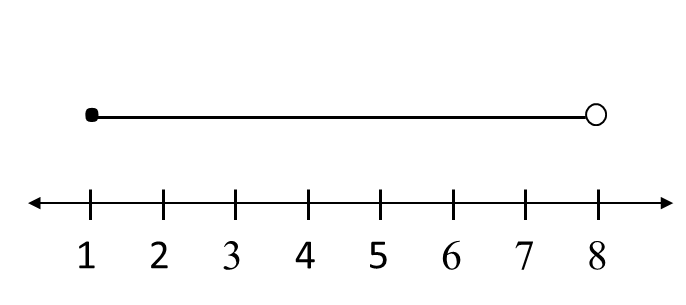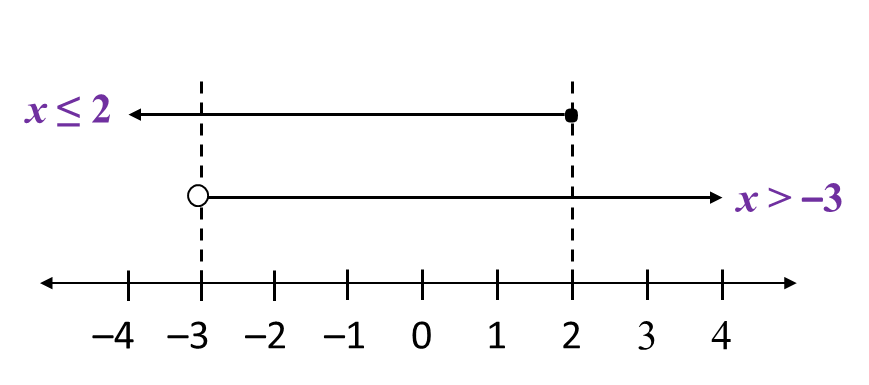# 7.2.1 Linear Inequalities, PT3 Focus Practice

                 
         
7.2.1 Linear Inequalities, PT3 Focus Practice
          

         Question 1:
          
Draw a number line to represent the solution for the linear inequalities –3 < 5 – x  ≤ 4.
          

          
Solution:
          
–3 < 5 – x   and   5 – x  ≤ 4
          
x < 5 + 3   and   x  ≤ 4 – 5
          
x < 8   and   x ≤ –1 → x  ≥ 1
         
          

          Thus, the solution is 1 ≤ x < 8Question 2:
          
Solve the following simultaneous linear inequalities.
          
          $3x-5\le 1\text{and}2-\frac{1}{3}x<3$              
          

          
Solution:
          
3x – 5 ≤ 1
          
3x  ≤ 1 + 5
          
3x  ≤ 6
          
x  ≤ 2The solution is –3 < x  ≤ 2.
       
       
  
  

         
Question 3:
          
The solution for the inequality 2 + < 3x – 4 is
          

          
Solution:
          
2 + x < 3x – 4
          
x – 3x < –4 – 2
          
–2x < –6
          
x < –3
          
x > 3
       
       
  
  
                 
         
Question 4:
          
The solution for the inequality –2 (6y + 3) < 3 (4 – 2y) is
          

         
          
Solution:
          
–2 (6y + 3) < 3 (4 – 2y)
          
–12y – 6 < 12 – 6y
          
–12y + 6< 12 + 6
          
–6y < 18
          
y < 3
          
y > –3